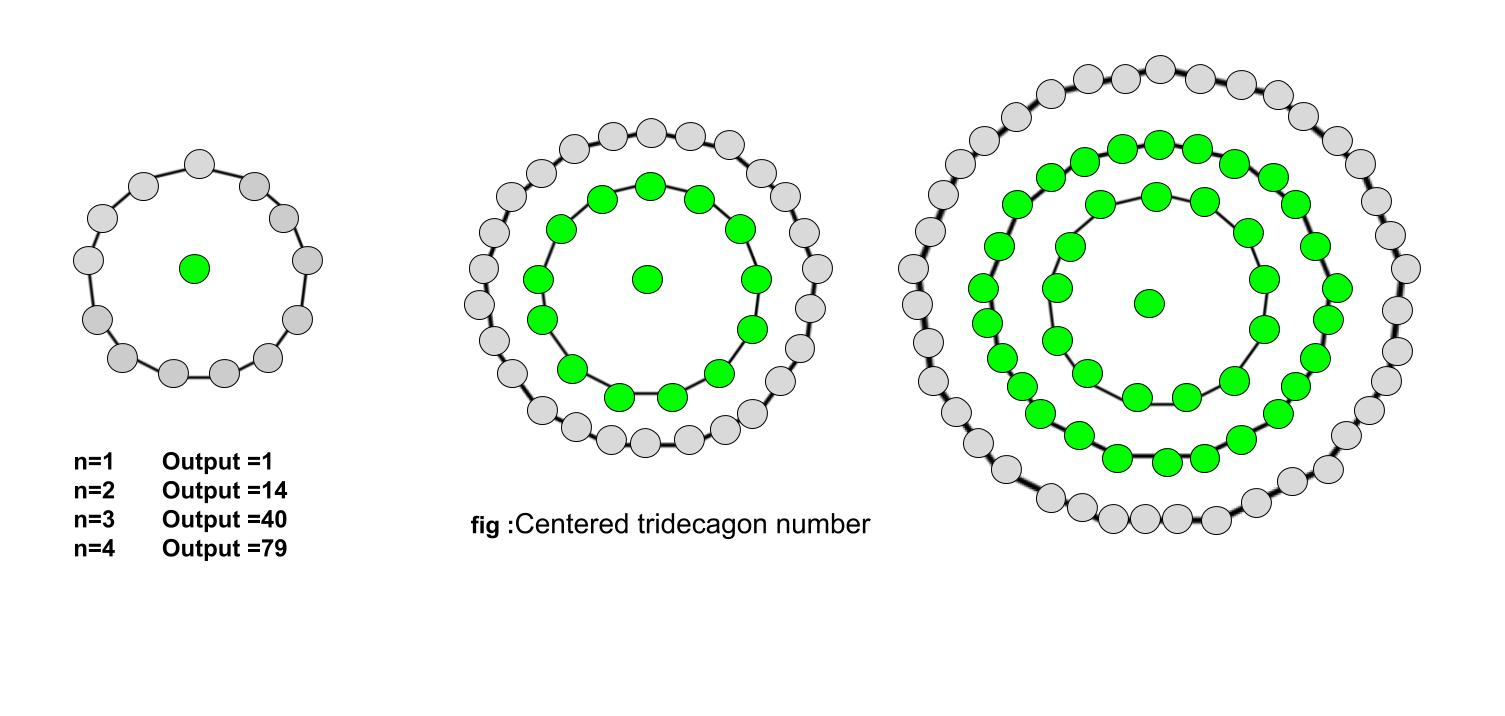Related Articles

# Centered tridecagonal number

• Last Updated : 16 Jul, 2021

Given a number n, the task is to find the nth Centered Tridecagonal Number.
A Centered tridecagonal number represents a dot at the center and other dots surrounding the center dot
in the successive tridecagonal(13 sided polygon) layer.

Examples :

Input : 2
Output : 14

Input : 9
Output : 469Formula for nth Centered tridecagonal number:

``

## C++

 `// C++ Program to find nth``// centered tridecagonal number``#include ``using` `namespace` `std;` `// Function to find nth centered``// tridecagonal number``int` `centeredTridecagonalNum(``long` `int` `n)``{``    ``// Formula to calculate nth``    ``// centered tridecagonal number``    ``return` `(13 * n * (n - 1) + 2) / 2;``}` `// Drivers code``int` `main()``{``    ``long` `int` `n = 3;``    ``cout << centeredTridecagonalNum(n);``    ``cout << endl;``    ``n = 10;``    ``cout << centeredTridecagonalNum(n);` `    ``return` `0;``}`

## Java

 `// Java Program to find nth``// centered tridecagonal number``import` `java.io.*;` `class` `GFG``{` `// Function to find nth centered``// tridecagonal number``static` `long` `centeredTridecagonalNum(``long` `n)``{``    ``// Formula to calculate nth``    ``// centered tridecagonal number``    ``return` `(``13` `* n * (n - ``1``) + ``2``) / ``2``;``}` `// Driver Code``public` `static` `void` `main (String[] args)``{``    ``long` `n = ``3``;``    ``System.out.println(centeredTridecagonalNum(n));``    ``n = ``10``;``    ``System.out.println(centeredTridecagonalNum(n));``}``}` `// This code is contributed by anuj_67.`

## Python3

 `# Program to find nth centered``# tridecagonal number` `# Function to find centered``# tridecagonal number``def` `centeredTridecagonalNum(n) :``    ` `    ``# Formula to calculate nth``    ``# centered tridecagonal number``    ``return` `(``13` `*` `n ``*``           ``(n ``-` `1``) ``+` `2``) ``/``/` `2` `# Driver Code``if` `__name__ ``=``=` `'__main__'` `:``        ` `    ``n ``=` `3``    ``print``(centeredTridecagonalNum(n))``    ``n ``=` `10``    ``print``(centeredTridecagonalNum(n))``                ` `# This code is contributed``# by akt_mit`

## C#

 `// C# Program to find nth``// centered tridecagonal number``using` `System;` `class` `GFG``{` `// Function to find nth centered``// tridecagonal number``static` `long` `centeredTridecagonalNum(``long` `n)``{``    ``// Formula to calculate nth``    ``// centered tridecagonal number``    ``return` `(13 * n * (n - 1) + 2) / 2;``}` `// Driver Code``public` `static` `void` `Main ()``{``    ``long` `n = 3;``    ``Console.WriteLine(centeredTridecagonalNum(n));``    ``n = 10;``    ``Console.WriteLine(centeredTridecagonalNum(n));``}``}` `// This code is contributed by anuj_67.`

## PHP

 ``

## Javascript

 ``
Output :
```40
586```

Time Complexity: O(1)
Auxiliary Space: O(1)

Reference: http://oeis.org/wiki/Figurate_numbers

Attention reader! Don’t stop learning now. Get hold of all the important DSA concepts with the DSA Self Paced Course at a student-friendly price and become industry ready.  To complete your preparation from learning a language to DS Algo and many more,  please refer Complete Interview Preparation Course.

In case you wish to attend live classes with experts, please refer DSA Live Classes for Working Professionals and Competitive Programming Live for Students.

My Personal Notes arrow_drop_up